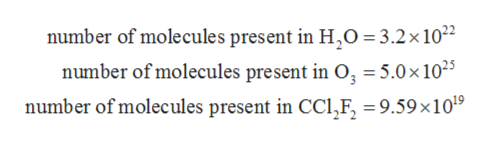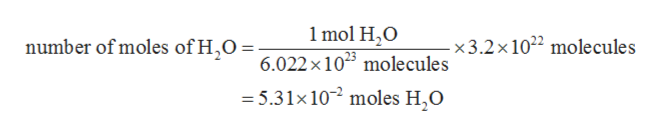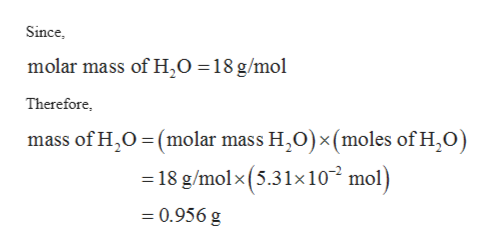# Calculate the mass (in g) of each sample. 3.2x10^22 H2O molecules 5.0x10^25 O3 molecules 9.59x10^19 CCl2F2 molecules

Question
92 views

Calculate the mass (in g) of each sample.

3.2x10^22 H2O molecules

5.0x10^25 O3 molecules

9.59x10^19 CCl2F2 molecules

check_circle

Step 1

The values are given as follows:help_outlineImage Transcriptionclosenumber of molecules present in H,O 3.2x 102 number of molecules present in O, = 5.0 x 1025 number of molecules present in CCl,F, =9.59x1019 fullscreen
Step 2

Since, Avogadro’s number is equal to 6.022 x 1023 molecules in a mole of a substance. Therefore, number of moles of H2O can be calculated with the help of Avogadro’s number as follows:help_outlineImage Transcriptionclose1 mol H2O number of moles of H,6.022 x 105 molecules - x3.2 x 1022 molecules = 5.31x 10 moles H,O fullscreen
Step 3

The mass of H2O can be calculated with the help of mo...help_outlineImage TranscriptioncloseSince molar mass of H,0 = 18 g/mol Therefore, mass of H2O (molar mass H,O)x (moles of H,O) 18 g/molx (5.31x102 mol) 0.956 g fullscreen

### Want to see the full answer?

See Solution

#### Want to see this answer and more?

Solutions are written by subject experts who are available 24/7. Questions are typically answered within 1 hour.*

See Solution
*Response times may vary by subject and question.
Tagged in
ScienceChemistry

### Physical Chemistry